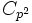# Classification of groups of prime-square order

## Statement

Let$p$ be a prime number. Then there are, up to isomorphism of groups, only two groups of order$p^2$:

• cyclic group of prime-square order, i.e., the cyclic group of order$p^2$, denoted$C_{p^2}$ or$\mathbb{Z}_{p^2}$.
• elementary abelian group of prime-square order, i.e., the elementary abelian group of order$p^2$, denoted$E_{p^2}$ and equal to$\mathbb{Z}_p \times \mathbb{Z}_p$. In the case$p = 2$, this is more commonly called the Klein four-group.

Note that both of these are abelian groups, so in particular we see that any prime-square is an abelianness-forcing number.

For detailed information comparing the two groups side by side, see groups of prime-square order

## Facts used

The table below lists key facts used directly and explicitly in the proof. Fact numbers as used in the table may be referenced in the proof. This table need not list facts used indirectly, i.e., facts that are used to prove these facts, and it need not list facts used implicitly through assumptions embedded in the choice of terminology and language.
Fact no. Statement Steps in the proof where it is used Qualitative description of how it is used What does it rely on? Difficulty level Other applications
1 Prime power order implies not centerless Step (1) Eliminates the case of trivial center class equation of a group 3 click here
2 Center is normal Step (2) Justifies taking the quotient by the center 0 click here
3 Cyclic over central implies abelian Step (2) Rules out the possibility of a center of order$p$, since the quotient would then be forced to be cyclic click here
4 Lagrange's theorem Steps (2), (3) Narrows down possibilities for order of center, and allows for computation of order of quotient group 2 click here
5 Equivalence of definitions of group of prime order Step (2) Show cyclicity of$P/Z$ if$Z$ has order$p$ click here
6 Structure theorem for finitely generated abelian groups Classifying the abelian groups click here

## Proof

The proof has two parts. First, we show that any group of order$p^2$ must be an abelian group. Then, we use the structure theorem for finitely generated abelian groups to argue that there are only two possibilities.

### Proof that the group must be abelian

This proof uses a tabular format for presentation. Provide feedback on tabular proof formats in a survey (opens in new window/tab) | Learn more about tabular proof formats|View all pages on facts with proofs in tabular format

Given: A prime number$p$, a group$P$ of order$p^2$.

To prove:$P$ is abelian.

Proof: Let$Z$ be the center of$P$. Our goal is to show that$Z = P$.

Step no. Assertion/construction Facts used Given data used Previous steps used Explanation
1$Z$ is nontrivial Fact (1)$P$ is nontrivial and its order is a power of a prime. -- --
2 The order of$Z$ cannot be$p$ Facts (2), (3), (4), (5)$P$ has order$p^2$ -- [SHOW MORE]
3 The order of$Z$ must be$p^2$ Fact (4)$P$ has order$p^2$ Steps (1), (2) [SHOW MORE]
4$Z = P$, so$P$ is abelian Step (3) Follows directly.

### Classification of abelian groups

This follows directly from fact (6) -- the group must be a direct product of cyclic groups, giving precisely the two possibilities mentioned.